# Solving MultiStep Equations Warm Up Solve 1 3

• Slides: 20Solving Multi-Step Equations Warm Up Solve. 1. 3 x = 102 x = 34 y 2. 15 = 15 y = 225 3. z – 100 = 21 z = 121 4. 1. 1 + 5 w = 98. 6 w = 19. 5Solving Multi-Step Equations Essential Question: How do you solve multi-step equations? Standards: MCC 8. EE. 7 Solve linear equations in one variable.Solving Multi-Step Equations Steps 1. Draw a line down the equal sign to separate the two sides of the equation. 2. Change all subtractions by adding a line and changing the sign. 3. Distribute if needed. 4. Combine Like Terms 5. Solve the equation by doing inverse operations.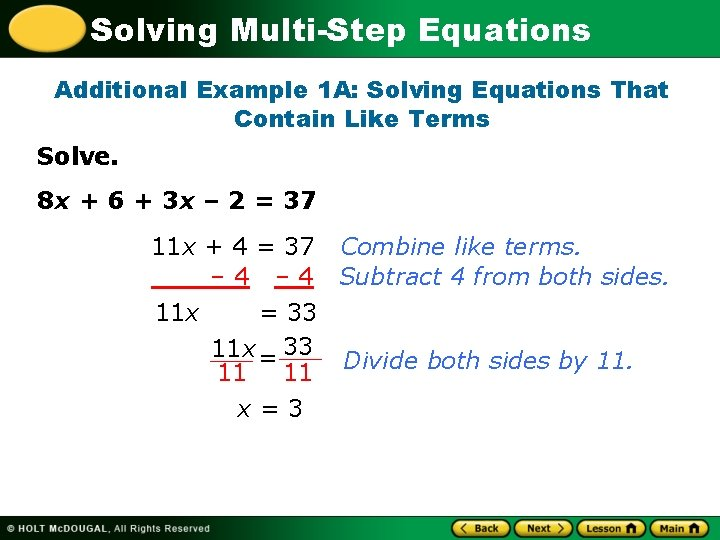Solving Multi-Step Equations Additional Example 1 A: Solving Equations That Contain Like Terms Solve. 8 x + 6 + 3 x – 2 = 37 11 x + 4 = 37 Combine like terms. – 4 Subtract 4 from both sides. 11 x = 33 Divide both sides by 11. 11 11 x=3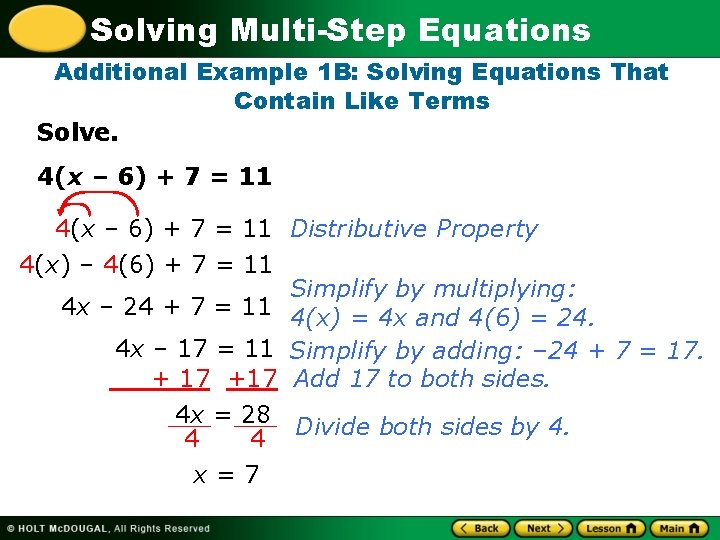Solving Multi-Step Equations Additional Example 1 B: Solving Equations That Contain Like Terms Solve. 4(x – 6) + 7 = 11 Distributive Property 4(x) – 4(6) + 7 = 11 Simplify by multiplying: 4 x – 24 + 7 = 11 4(x) = 4 x and 4(6) = 24. 4 x – 17 = 11 Simplify by adding: – 24 + 7 = 17. + 17 +17 Add 17 to both sides. 4 x = 28 Divide both sides by 4. 4 4 x=7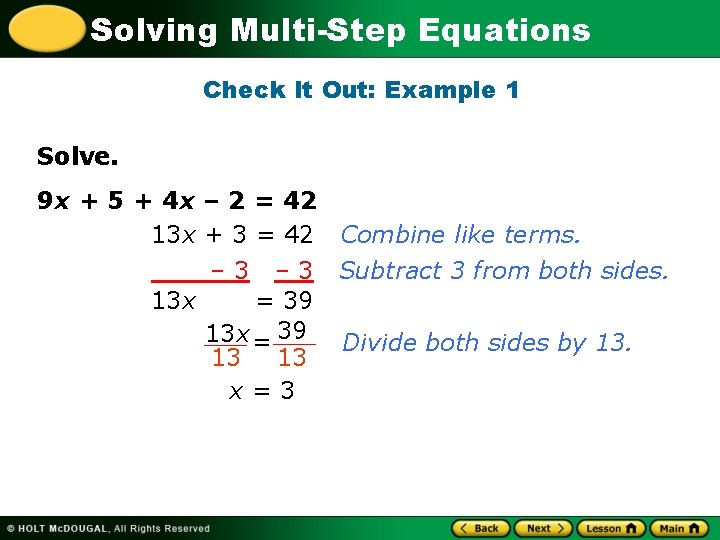Solving Multi-Step Equations Check It Out: Example 1 Solve. 9 x + 5 + 4 x – 2 = 42 13 x + 3 = 42 Combine like terms. – 3 Subtract 3 from both sides. 13 x = 39 Divide both sides by 13. 13 13 x=3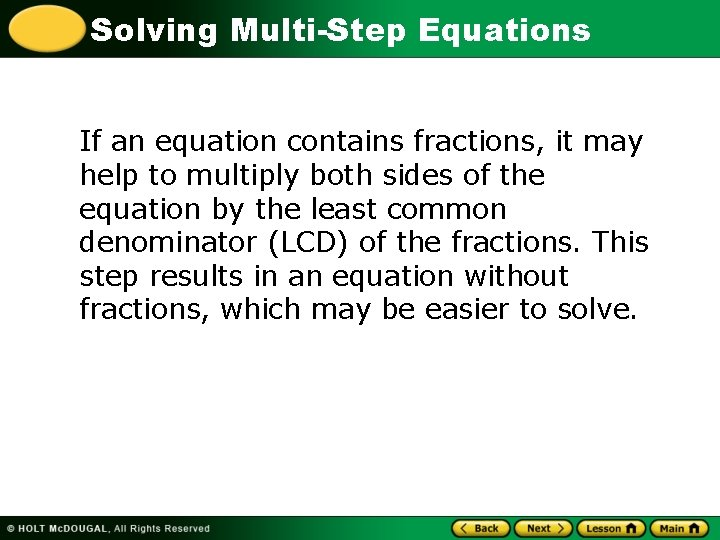Solving Multi-Step Equations If an equation contains fractions, it may help to multiply both sides of the equation by the least common denominator (LCD) of the fractions. This step results in an equation without fractions, which may be easier to solve.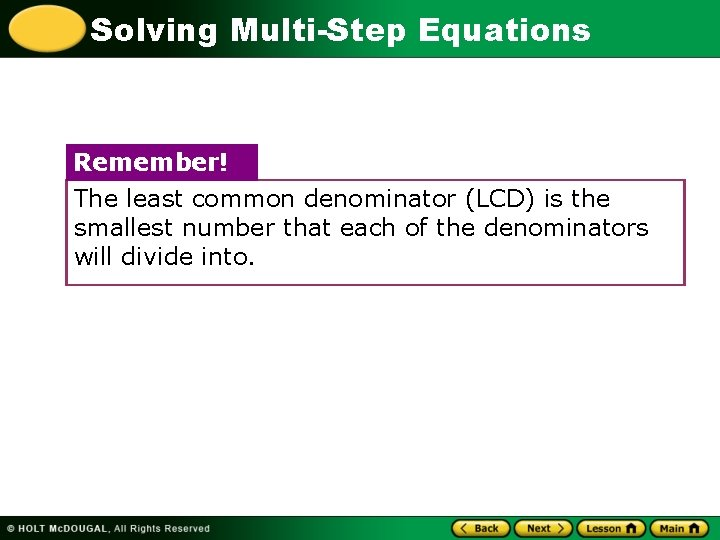Solving Multi-Step Equations Remember! The least common denominator (LCD) is the smallest number that each of the denominators will divide into.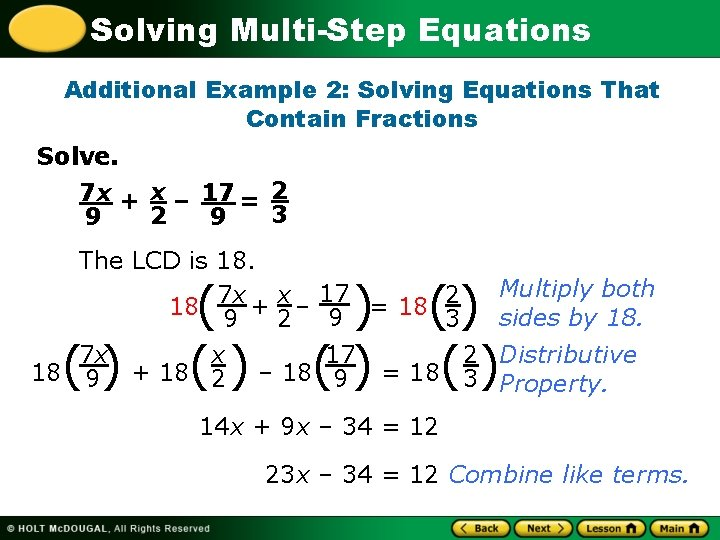Solving Multi-Step Equations Additional Example 2: Solving Equations That Contain Fractions Solve. 7 x + x – 17 = 2 3 2 9 9 The LCD is 18. Multiply both 17 7 x x 2 18 + – 9 = 18 sides by 18. 9 3 2 7 x x 17 2 Distributive 18 9 + 18 2 – 18 9 = 18 3 Property. () ( () ) () () () 14 x + 9 x – 34 = 12 23 x – 34 = 12 Combine like terms.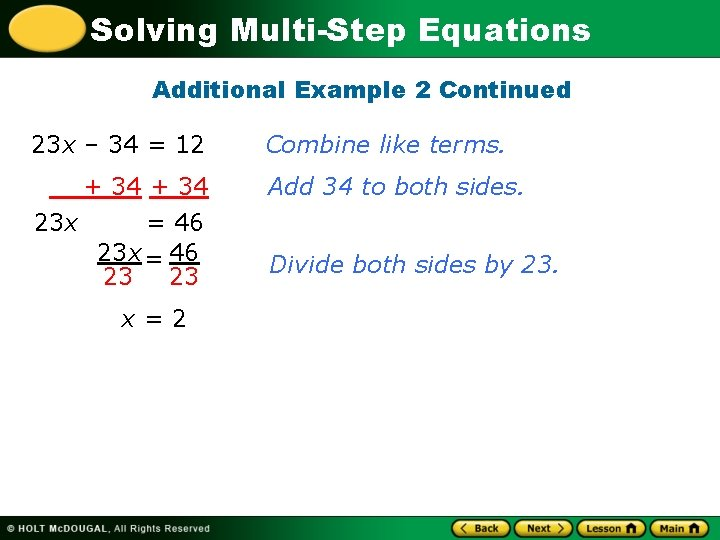Solving Multi-Step Equations Additional Example 2 Continued 23 x – 34 = 12 Combine like terms. + 34 23 x = 46 23 23 Add 34 to both sides. x=2 Divide both sides by 23.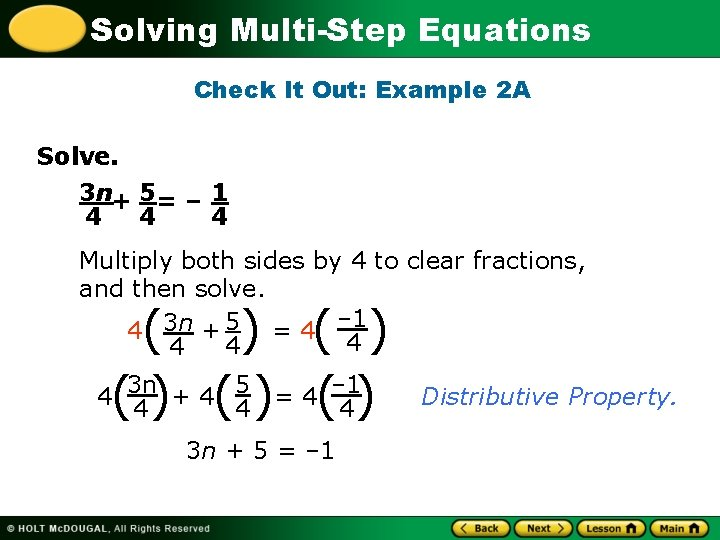Solving Multi-Step Equations Check It Out: Example 2 A Solve. 3 n+ 5= – 1 4 4 4 Multiply both sides by 4 to clear fractions, and then solve. – 1 4 3 n + 5 = 4 4 ( ) 5 = 4 – 1 4(3 n + 4 (4) ( 4) 4) 3 n + 5 = – 1 Distributive Property.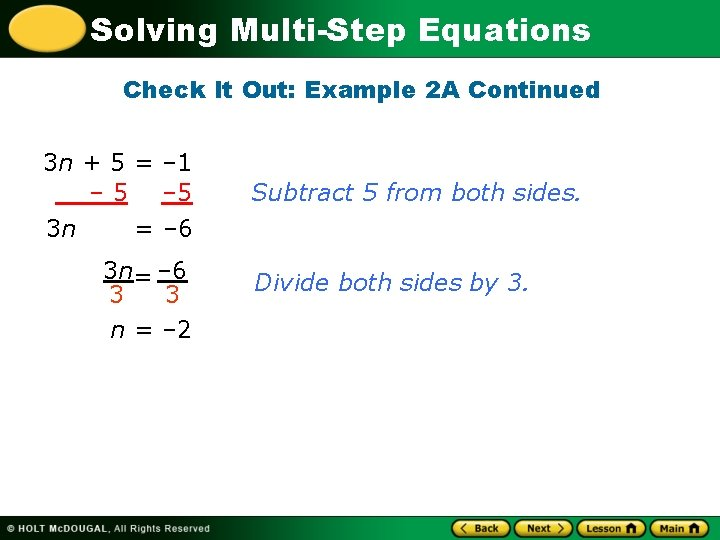Solving Multi-Step Equations Check It Out: Example 2 A Continued 3 n + 5 = – 1 – 5 3 n = – 6 3 n= – 6 3 3 n = – 2 Subtract 5 from both sides. Divide both sides by 3.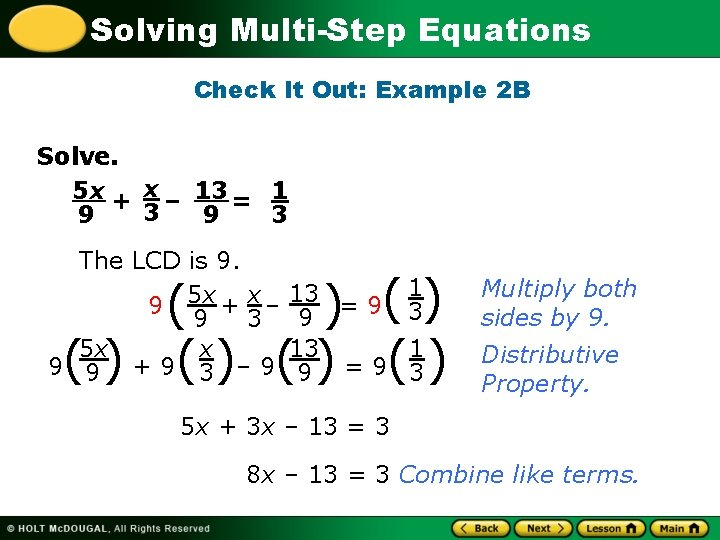Solving Multi-Step Equations Check It Out: Example 2 B Solve. 5 x + x – 13 = 1 3 9 9 3 The LCD is 9. 9 5 x + x – 9 3 5 x x 9 9 +9 3 – 9 ( ) () () () 13 = 9 1 3 9 13 1 9 =9 3 Multiply both sides by 9. Distributive Property. 5 x + 3 x – 13 = 3 8 x – 13 = 3 Combine like terms.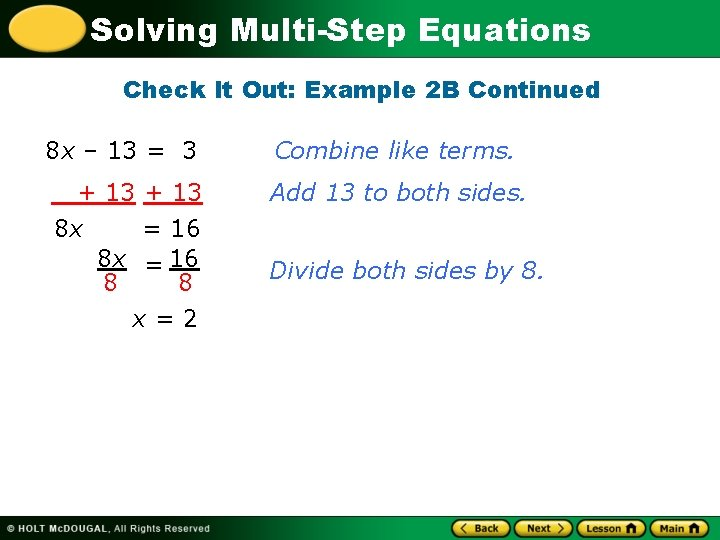Solving Multi-Step Equations Check It Out: Example 2 B Continued 8 x – 13 = 3 Combine like terms. + 13 8 x = 16 8 8 x=2 Add 13 to both sides. Divide both sides by 8.Solving Multi-Step Equations Check It Out: Example 2 B Continued Check 5 x + x – 13 = 1 3 3 9 9 5(2) 13 ? 1 Substitute 2 for x. 9 + 3 – 9 =3 10 2 13 ? 1 9 +3 – 9 =3 ? 3 10 + 6 – 13 = The LCD is 9. 9 9 ? 3 3= 9 9Solving Multi-Step Equations Pg. 115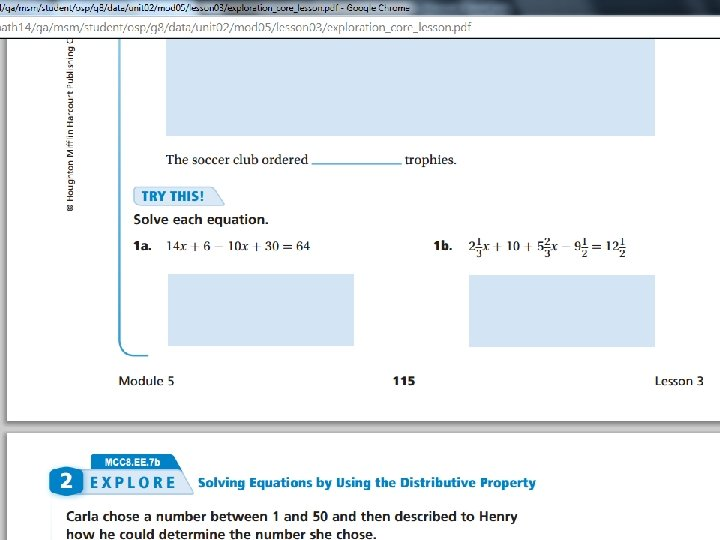Solving Multi-Step EquationsSolving Multi-Step Equations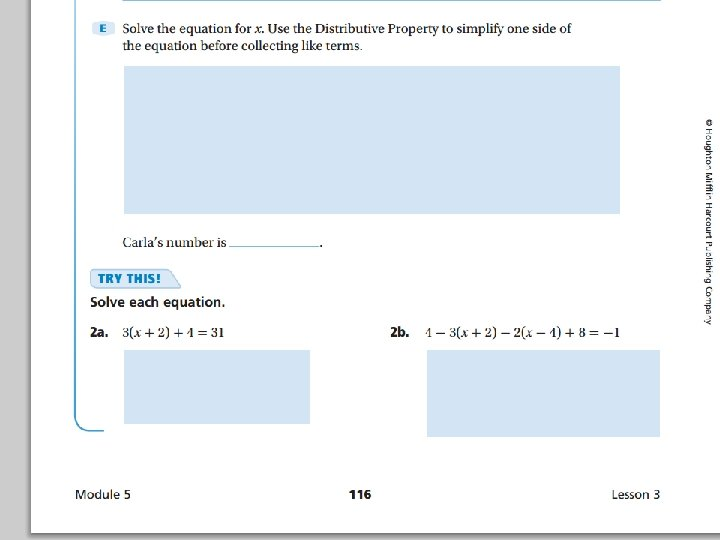Solving Multi-Step EquationsSolving Multi-Step Equations #1 -10 check answers by scanning the QR code